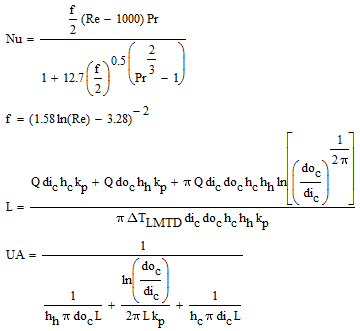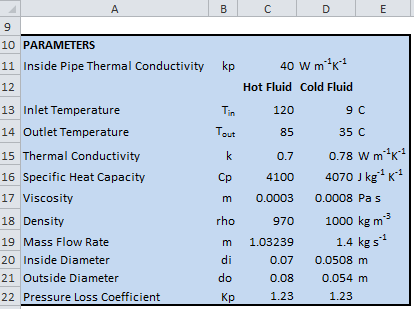## Monday, June 10, 2019

This Excel spreadsheet helps you design a double pipe heat exchanger (also known as a concentric tube heat exchanger). Double pipe heat exchangers are often used in the chemical, food processing and oil & gas industries. They have a particular advantage when close temperature approaches are needed or in high pressure applications.

The spreadsheet will give you important design parameters, such as the overall heat transfer area, the length required, pressure drops, fluid velocities and much more. The spreadsheet uses the

Gnielinski correlation for the heat transfer coefficient of the shell side and tube side fluids (hh and hc). The Gnielinski correlation is valid for 0.5 ≤ Pr ≤ 2000 and 2300 ≤ Re ≤ 56 .It gives the heat transfer coefficient in terms of the friction factor, the Reynolds number and the Prandtl number.
Filonenko correlation for the friction factor (valid for smooth pipes in turbulent flow with 104 ≤ Re ≤ 107).

The equations for the heat transfer coefficients, the friction factor, the length of the heat exchanger and the overall heat transfer coefficient are given below• Nu is the Nusselt Number
• Re is the Reynolds Number
• f is the friction factor
• Q is the rate of heat transfer (determined from a heat balance)
• dic is the internal diameter of the inner pipe
• doc is the external diameter of the inner pipe
• kp is the thermal conductivity of the pipe material
• hc is the heat transfer coefficient of the cold fluid (in the inner pipe)
• hh is the heat transfer coefficient of the hot fluid (in the outer pipe)
• ΔTLMTD is the log mean temperature difference.
The Excel spreadsheet uses variable names in the formulas to help you better understand the equations. Generally, the suffixes h and c represents quantities defined for the hot and cold streams respectively (e.g. hh is the heat transfer coefficient for the hot stream, hc is the heat transfer coefficient for the cold stream).INSTRUCTIONS :-

Step 1. Enter your parameters. The spreadsheet will calculate initial values of the heat transfer coefficients, the rate of heat transfer across both fluids, and the length of the heat exchanger. Provide an initial guess for the parameter (i.e. mass flowrate) you will change in Step 2.Specify your parameters

Step 2. Equalize heat transfer across both streams. The rate of heat transfer across the shell side and tube side fluids must be the same. You must use Goal Seek to make the difference between the two rates of heat transfer equal to zero by changing a design parameter (e.g. mass flowrates)Goal Seek

You have now established important design parameters, such as the fluid velocities, the tube-side and shell-side pressure drops, Prandtl numbers, the length of the heat exchanger and so on.Intermediate Calculations and Results